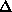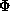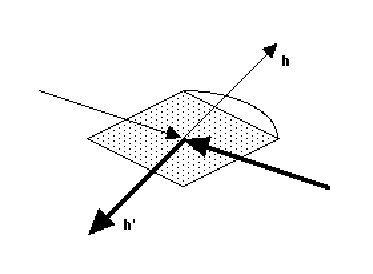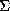Philip R. Evans,
MRC Laboratory of Molecular Biology, Hills Road, Cambridge CB22QH

The integrated intensities from any data collection experiment are not all on the same scale, because of various systematic differences in the collection procedure. It is the task of the "data reduction" protocol to place all observations on a common scale, to detect and reject outliers (reflections for which the data collection has gone badly wrong), and to produce a list of |F| and(|F|) for the structure determination. There are some special considerations in the optimum treatment of data intended for MAD phasing, in that we want very accurate differences between amplitudes, for the anomalous differencesF+/- and the dispersive differencesF, rather than the most accurate absolute values. This means a difference both in data collection strategy, designing the experiment to minimize the systematic errors in the differences, and in the scaling strategy, in which relative scaling can reduce, though probably not eliminate, the systematic errors. In the MAD phasing method, we need accurate differences because the small signal is easily swamped by systematic errors, and we also need to be careful about eliminating outliers, since a small number of spurious large differences can confuse both Patterson and direct methods of locating the anomalous diffracting centres.

To aid designing data collection and scaling strategies, it is helpful to enumerate the reasons for the observed intensities not being on the same scale. These factors can be roughly divided into those that can be in principle calculated, and those that must be determined empirically from the data.

(1) Calculable scale factors

* Lorentz factor - this is uncertain close to the rotation axis, but is not normally a problem

* Polarization - this may be uncertain for synchrotron radiation, but the error is small

* Corrections arising from deficiencies in the integration program - if the geometrical parameters used by the integration program are inaccurate, the prediction of which spots are partially recorded will also be inaccurate. The estimated partiality may be improved by post-refinement (eg in Scalepack or Mosflm)

* Different truncation of the tails of reflections caused by diffuse scattering - partially recorded reflections are measured over at least twice the rotation range of fully recorded reflections, so if the spots have long tails in the rotation direction, more of the tails will be included in partials than in fulls (the TAILS correction in Scala is an attempt to correct for this, see appendix below).

(2) Empirical scale factors

These are usually subsumed into general scaling.

* Change of incident beam intensity - mainly on synchrotrons

* Change of detector sensitivity - the variation of sensitivity across the detector is best determined in a separate calibration (flood-field correction), but the overall "sensitivity" may be taken up in the scaling, particularly for film or off-line image plates

* Different crystals

* Illuminated volume - if the crystal is larger than the beam. This is indistinguishable from absorption in the incident beam

* Absorption - less of a problem at short wavelengths, but hard to correct for satisfactorily

* Radiation damage - serious on unfrozen crystals

* Wavelength-dependent factors - mainly for the Laue method

It is possible to design the data collection strategy for MAD data collection such that many of these systematic errors can be made equal, so that they cancel out in the dispersive and anomalous differences. Note that this is the opposite of the optimum collection strategy for data intended for structure refinement, when ideally we should try to maximize the systematic differences between observations, so that the scaling procedure can determine the different corrections for different parts of the data, or least can average out the systematic errors.

(a) Dispersive differences (ie between different wavelengths) - measurements of the same reflection at different wavelengths will normally be made in the same way, so that the systematic arrors should be the same. The main difference is that they are necessarily measured at different times: radiation damage is the only difficult time-dependent scale, hence the great advantage of using frozen crystals. On unfrozen crystals, the strategy must be to collect different wavelengths close together in time (eg as images interleaved at each wavelength).

(b) Anomalous differences - it is not possible to collect I+ and I- in exactly the same way, on the same area of the detector. The most difficult correction is absorption, other corrections are likely to be the same. Absorption is a serious problem at the longer-wavelength edges (eg Fe), less of a problem for Se or Br edges.

There are two ways of minimizing the absorption differences, though neither will eliminate the problem:-

(i) inverse beam method - measure reflections atand+180deg.. This inverts the direction of the incident and diffracted beams. The absorption will only be the same if the crystal and its mount have a centre of symmetry(i)(ii)

(ii) rotate the crystal about a two-fold axis, and collect Bijvoet pairs (eg hkl, hk-l for a crystal rotating around the c axis) on the same image. This requires the crystal to be aligned about an axis, at least approximately. The absorption is only the same if the crystal and its mount have a plane of symmetry perpendicular to the rotation axis.

To correct for absorption differences between Bijvoet pairs, the scaling model must be able to apply a different scale to I+ and to I-, so the scaling model must be anisotropic and non-centrosymmetric. Suitable functions are 3-dimensional smoothed scales (local scales) and 3D functions such as spherical harmonics. The functions must not vary too much locally, otherwise the real differences will be scaled out.

How well are scale factors determined?

The problem with 3-dimensional scale functions is that they are typically ill-determined by the observed data. The empirical correction factors listed above may be divided into two categories:-

1) functions of the incident beam direction (illuminated volume, absorption in the incident beam) or of time,which is equivalent (beam intensity, radiation damage). With any area detector, these functions are well-determined, since many reflections are measured at the same time for each direction.

2) functions of the diffracted beam direction (absorption, radial dependence of radiation damage). These functions are poorly determined, since there are relatively few observations in each direction. The corrections are well-determined only:-

(a) with high symmetry (thus high redundancy of measurements made under different conditions)

(b) collection by rotation about more than one axis (to measure equivalent reflections with different beam paths in the crystals)

(c) scaling relative to a reference set - this gives relative rather than absolute scales, but is useful to reduce systematic errors in differences, as is required for MAD data.

A relative-scaling protocol

The following suggested protocol for scaling MAD data uses a reference data set, which provides a an anchor for the scaling parameters. Note that in the reference, I+ and I- are averaged, so that in the real datasets, systematic bias in the anomalous difference will be reduced (the meanI+/- should be zero). A similar protocol is also useful for scaling heavy-atom derivatives using the native dataset as reference, in the MIR method.

1. Choose reference set: this should be (in order of importance)

(a) the most complete

(b) the most accurate

(c) remote from the anomalous edge

2. Scale and merge the reference set, merging I+ and I-, to get a unique set of merged intensities Iref

3. Sort the reference set together with all unmerged data, for all wavelengths (including the set used as reference, if this is to be used in phasing).

4. scale all data together, perhaps in two passes

(a) batch scaling (scale k & B-factor for each image ("batch")) to remove discontinuities between images. If all images are reliably on a similar scale with no discontinuities between images (stable source, collected by dose etc), this step may be omitted.

(b) smooth scaling using a 3-dimensional anisotropic or local scaling model. This may be parameterized in camera space (x,y,or beam directions) or in crystal space (h,k,l). An example in Scala would be SCALES ROTATION SPACING 10 DETECTOR 3 3.

5. split out each wavelength, either averaging repeated and symmetry-related observations, or keeping them separate (depending on whether the phasing strategy uses merged or unmerged data)

Various programs allow scaling of this type, eg the XDS package (Kabsch 1988)), the CCP4 program SCALA (which took some inspiration from the Kabsch method), and X-GEN (Howard)

Results

Trials with a Se-methionine data set (thanks to Richard Pauptit) and a Br-uridine DNA set (thanks to Harry Powell and Christine Cardin) showed a small but significant improvement using this protocol, compared to scaling each dataset separately. The improvement is presumably only small because absorption, which causes the most serious systematic errors is small at the Se and Br edges. Absorption is much more serious at longer wavelength, so for MAD measurements on for example the Fe edge this scaling method would produce a much larger gain. However, since the MAD signal is so small, even a small improvement can make the difference between success and failure, and a small reduction in the difference between observations (as measured by reduced dispesive and anomalous differences) may make a substantial difference in phasing.

Appendix

A simple correction for the bias between fully-recorded and partially-recorded reflections caused by diffuse scattering

Many protein crystals show marked diffuse scattering, which is seen as long tails on spots in the "phi" direction, so that reflections often appear on the image before they are predicted. If the mosaicity is increased to include these tails, too many reflections may be rejected as overlaps. Fully-recorded reflections are integrated over a smaller phi width than partials, so more of the tails are chopped off for fulls than for partials. This leads to the typical negative partial bias, with partials systematically larger than equivalent fulls.

A correction has been introduced into SCALA which attempts to correct for the different truncation of diffuse scattering tails, using a simple model of thermal diffuse scattering, expressed as 2 or 3 parameters over the whole data set. This implementation does not attempt to correct for diffuse scattering itself, only for the different effect on fulls and partials. This correction reduces the partial bias substantially, and seems to improve the data generally, though sometimes the parameter refinement can be a little unstable.

The method

This algorithm was inspired by the correction described by Blessing (1987), but in his case full profiles of the diffraction spots were analysed to determine the diffuse scattering contribution. Data collected with relatively coarse rotation slices do not provide enough information to do this, and the typical crowded diffraction patterns of macromolecule crystals make it harder to extract full profiles, since the spots may overlap.

1. The thermal diffuse scattering contribution to the integrated intensity is proportional to the Bragg intensity J. If the complete profile is measured, the measured intensity I, including diffuse scatter is given by

I = J (1+)

whereis a proportionality constant

2. The proportionality constantvaries with resolution, and may be anisotropic. At present an isotropic model is implemented in Scala=0 + s21

where s2 = (sin/)2, and0 is normally = 0.0 and1 are refinable parameters.

3. The width of the thermal diffuse scattering peak is assumed to be constant in reciprocal space, = v, a refinable parameter. The distance in reciprocal space travelled by a reflection rotated by an angleat a radiusfrom the rotation axis is given by

q =4. The profile of the diffuse scattering peak is modelled as a triangle, with width v (in the reciprocal space coordinate q), and height h, where h is a function of, since the area of the triangle is I-J = h v = J, hence h = J/ v

5. If the scan width of an observation, including all parts of a partially recorded reflection, is less than 2v, the tails of the diffuse scattering peak may be truncated, clipping off areas C1 and C2 (>= 0) (see figure). These areas may be calculated from the rotation angles at the start of the scan (the beginning of the first image contributing to the observation), the centre of the reflection (the predicted angle), and the end of the scan (the end of the last image contributing to the observation).6. The correction factor for diffuse scattering if the full profile were measured would be given by

J = I / (1 +)

For the truncated profile

J = I / (1 +( 1 - C1 - C2))

where C1 and C2 are expressed as fractions of the complete area of the triangle (h v)

Since I do not trust this simple formulation to correct properly for diffuse scattering, the correction used is

J = I (1 +) / (1 +( 1 - C1 - C2))

This corrects for the different truncation of the peak for different spots, particularly the difference between observations made over 1, 2 ,3 etc images, but not for the diffuse scattering itself.

The parameters refined are0,1 and v (note that C1 and C2 are functions of v), though normally0 is fixed at 0.0.

Results

Application of the Tails correction to to datasets with visible diffuse scattering typically has a dramatic improvement on the partial bias, ie the systematic difference between fully recorded and partially recorded reflections (see figure), and often a significant improvement in Rmerge. The correction is not well-determined if the diffuse scattering is small, nor if the mosaicity is badly underestimated in the integration process: in these cases, the parameters can take on unrealistic (eg negative) values.Example of the improvement in partial bias (lower curves) and Rmerge (upper curves), plotted against resolution. Solid lines: with Tails correction, dashed lines: without correction. The partial bias ish(<Ifull> - Ipartial) /h <Ifull>, where the summations are over all reflections for which there are both fulls and partials, <Ifull> is the mean of all the fully recorded observations of the reflection, and Ipartial is a summed partial observation

Acknowledgements: I thank Richard Pauptit, Harry Powell and Christine Cardin for the loan of datasets, Gérard Bricogne for helpful discussions on statistics, and Eric de la Fortelle for help in running SHARP.

References

Blessing, R.H. (1987), Data Reduction and Error analysis for Accurate Single Crystal Diffraction Intensities, Cryst. Rev. 1, 3-58

Howard, A.J. X-GEN documentation

Kabsch, W. (1988) Evaluation of single-crystal X-ray diffraction data from a position sensitive detector, J. Appl. Cryst. 21, 916-924